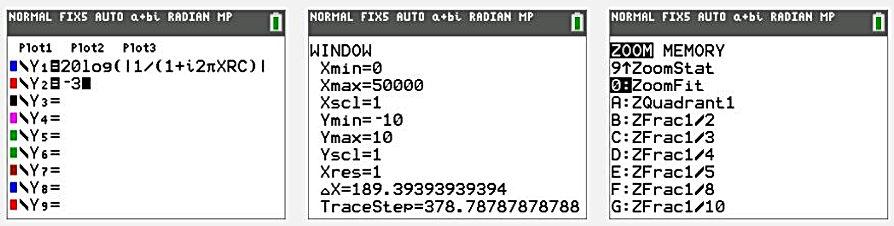naver-site-verification: naver47238a1b6bfbb19a2fd4b619734fa9a6.html
top of page

# Designing Filters with the Ti-84 plus (CE)

## Description

A method is shown to design electrical filters. Very useful for Electrical Engineering. As an example, we take a low pass filter consisting of a resistor of 1000 Ohm and a capacitor of 4,7 nF =0.00471uF. See the scheme.

The complex transfer function of this filter equals : Vout/Vin= 1/(1+i2πXR1C1) where X is the frequency (Hz) of the input Voltage. The value of R is stored in memory R, and the value of C is stored in memory C.

Using Y1 as the logarithmic absolute value of the transfer function for the Bode plot and Y2=-3 (dB) the Ti calculates the bandwidth (33782,44 Hz) by using the command intersect.#### Redesign

Next: Two examples are shown by using the numeric solver in the math menu to redesign the filter.

New bandwidth, 25000 Hz and capacitor 4.7nF results in a new resistor value of 1351.29 Ohm.

A bandwidth of 1000Hz and a resistor of 1000 Ohm results in a capacitor of 15.87nF.A bandwidth of 1000Hz  and a resistor of 1000 Ohm results in a capacitor of 15.87nFbottom of page